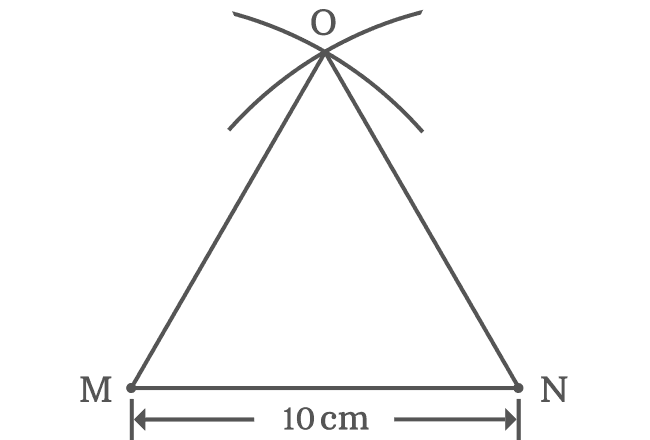# Construction of Equilateral triangle

An equilateral triangle can be constructed geometrically by using geometric tools. It is actually constructed by drawing sides of triangle with same length. The following animated example teaches you the geometrical procedure to construct an equilateral triangle.1. Consider a point in a plane and it is named as point $M$.
2. Take a ruler and draw a horizontal straight line of any length. In this example, $10 \, cm$ horizontal line is drawn and its end point is called as point $N$.
3. Take compass and set it to $10 \, cm$. Now, draw an arc from point $M$ and also draw another arc from point $N$. The two arcs are intersected at point $O$.

## Properties

Let’s investigate the basic properties of equilateral triangle from this constructed triangle.### Lengths

It is taken that the length of the side $\overline{MN}$ is $10 \, cm$ and also drawn arcs from points $M$ and $N$ with the same length.

Therefore, $MN = NO = OM$ $\,=\,$ $10 \, cm$

### Angles

Measure all three angles of this equilateral triangle by protractor. You will observe that,

$(1) \,\,\,$ $\angle MNO = 60^°$

$(2) \,\,\,$ $\angle NOM = 60^°$

$(3) \,\,\,$ $\angle OMN = 60^°$

It clears that all three angles of this triangle are equal and each angle is $\dfrac{\pi}{3}$. Therefore, it is proved that an equilateral triangle is also an equiangular triangle.

$\,\,\, \therefore \,\,\,\,\,\,$ $\angle MNO$ $\,=\,$ $\angle NOM$ $\,=\,$ $\angle OMN$ $\,=\,$ $60^°$

Latest Math Topics
Jun 26, 2023
Jun 23, 2023

###### Math Questions

The math problems with solutions to learn how to solve a problem.

Learn solutions

Practice now

###### Math Videos

The math videos tutorials with visual graphics to learn every concept.

Watch now

###### Subscribe us

Get the latest math updates from the Math Doubts by subscribing us.